# The King

The King wants to divide his sons equally. He has 42 rubies and 45 diamonds. How many sons and how will they share them?

n =  3
r =  14
d =  15

### Step-by-step explanation:

$r=42\mathrm{/}n=42\mathrm{/}3=14$
$d=45\mathrm{/}n=45\mathrm{/}3=15$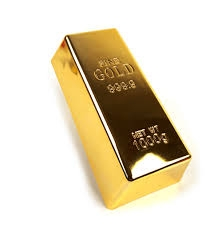Did you find an error or inaccuracy? Feel free to write us. Thank you!Tips to related online calculators
Do you want to calculate least common multiple two or more numbers?
Do you have a linear equation or system of equations and looking for its solution? Or do you have a quadratic equation?

## Related math problems and questions:

• PlumsIn the bowl are plums. How many would be there if we can divide it equally among 8, 10 and 11 children?
• Lcm simpleFind least common multiple of this two numbers: 140 175.
• LCM of two numberFind the smallest multiple of 63 and 147
• Gcd and lcm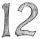Calculate the greatest common divisor and the least common multiple of numbers. a) 16 and 18 b) 24 and 22 c) 45 and 60 d) 36 and 30
• LCMWhat is the least common multiple of 5, 50, 14?
• Lcm of three numbersWhat is the Lcm of 120 15 and 5
• Trams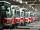Tram no. 3,7,10,11 rode together from the depot at 5am. Tram No. 3 returns after 2 hours, tram No. 7 an hour and half, no. 10 in 45 minutes and no. 11 in 30 minutes. For how many minutes and when these trams meet again?
• Plum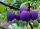On the platter are plum. How many were there if its have to be able to share equally among 8,10 and 12 children?
• Lcm = 22 + gcdThe least common multiple of two numbers is 22 more than their greatest common divisor. Find these numbers.
• DriversDriver Franta and driver Karel are friends. They leave the start-stop both at the same time in the afternoon - at 13.30. When will they meet again if Franta goes around his route in 50 minutes and Karel in 40 minutes?
• Dance groupThe dance group formed groups of 4, 5, and 6 members. Always one dancer remains. How many dancers were there in the whole group?
• Common divisorsFind all common divisors of numbers 30 and 45.
• PexesoDrawing paper has dimensions of 60cm and 840mm. Pupils have to divide it into squares so that they can make a pexeso. What dimension must squares have if their side is to be larger than 3cm and less than 10cm?
• Florist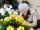Florist has 84 red and 48 white roses. How many same bouquets can he make from them if he must use all roses?
• Steps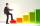Peter makes steps long 70 cm, John 45 cm long. After how many meters their footsteps meet?
• CherriesCherries in the bowl can be divided equally among 3 or 15 or 5 children. How many are the minimum cherries in the bowl?
• Bus linesAt 5.00 in the morning, four buses left the terminal at the same time. The first bus has an interval of 15 minutes, the second 20 minutes, the third 25 minutes, and the fourth 45 minutes. How many minutes will all four buses leave the terminal at the same